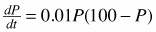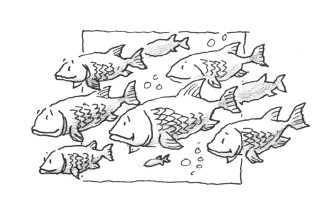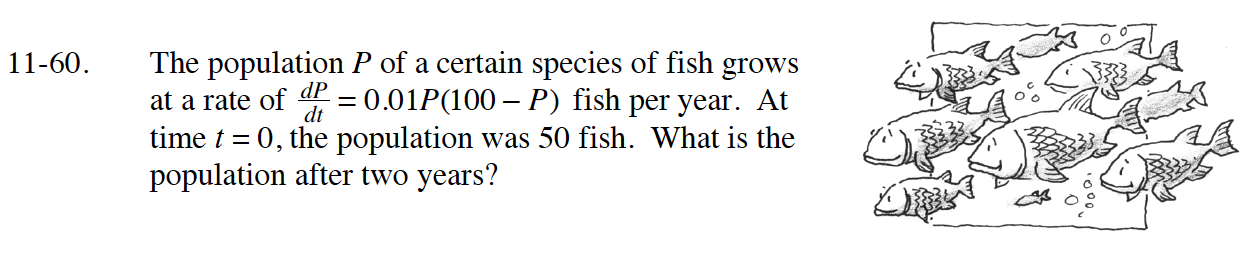### Home > CALC > Chapter 11 > Lesson 11.2.2 > Problem11-60

11-60.

The population P of a certain species of fish grows at a rate offish per year. At time t = 0, the population was 50 fish. What is the population after two years? Homework Help ✎$\frac{100dP}{P(100-P)}=dt$

$\int\frac{100dP}{P(100-P)}=\int dt$

When t = 0, P = 50. What is the value of C?

Use your value of C in your equation to determine the value of P when t = 2.